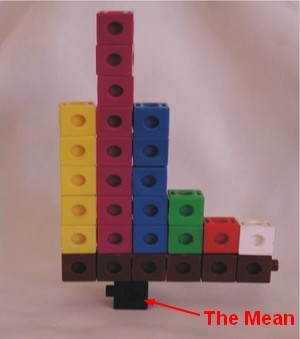# R - Summary

## Package Function

### Base

summary is a generic function used to produce result summaries of the results of various model fitting functions.

The function invokes particular methods which depend on the class of the object (ie first argument).

``````x = rnorm(50,5,4)
xSummary <- summary(x)
xSummary```
```
``````Min. 1st Qu.  Median    Mean 3rd Qu.    Max.
-4.963   3.434   5.706   5.800   8.119  16.140
```
```

For a data frame, you got to see the following for each variable:

Export it:

``````exportFile <- file("C:/temp/Myfile.tsv",open="w")
write.table(xSummary, sep="\t")
close(exportFile)```
```

### Psych

psych package

#### Total

``````# Load packages
library(psych)

# You can save the content of this function
mySummaryTable = describe(myData)```
```

#### By

##### describeBy

The describeBy function reports basic summary statistics by a grouping variable.

Useful if:

• the grouping variable is some experimental variable (ie dependent variable)
• the data are to be aggregated for plotting.

Partly a wrapper of the describe function.

An alternative function statsBy returns a list of means, n, and standard deviations for each group.

##### statsBy

statsBy is an alternative function an describeBy that returns a list of:

• n,

for each group.

### Dplyr

See also: R - Dplyr (Data Frame Operations) and the summarize function.

Discover MoreDistribution - (Mean|Average) (M| | )

The average is a measure of center that statisticians call the mean. To calculate the mean, you add all numbers and divide the total by the number of numbers (N). The mean is not resistant. The...R - Mean

This function calculate the mean. You can find it also in the summary statistics functionsR - Str (Structure)

Str display compactly the internal structure of an R object. Str is an alternative for summary where num: indicates the numeric class [1:5]: from one to 5 elements 1 -2 3.3 7 -3: the elements...